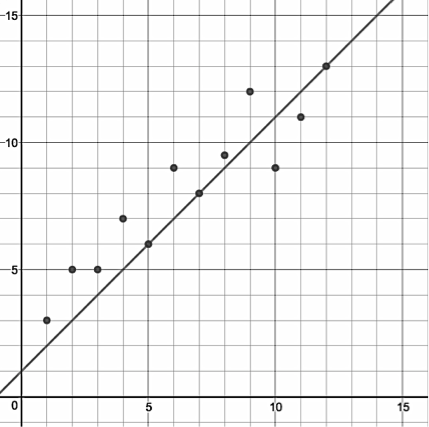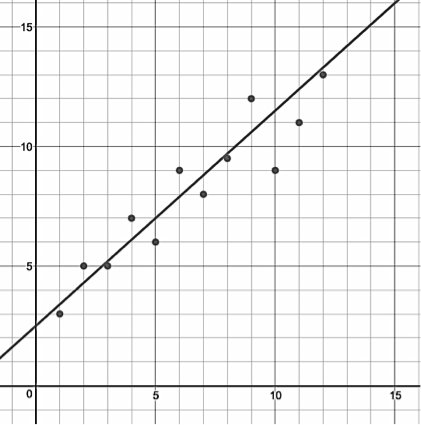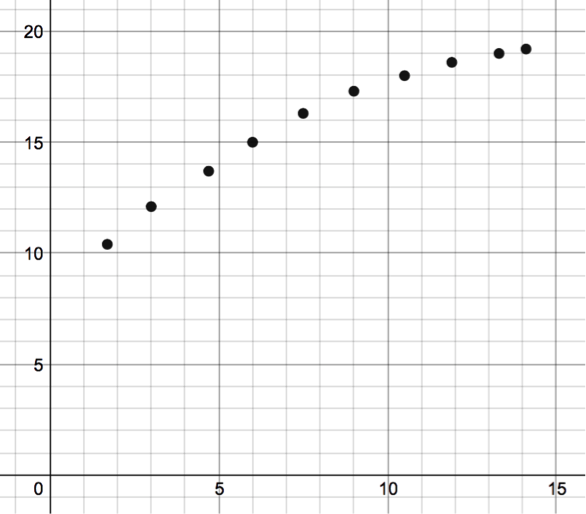# Descriptive Statistics

## Objective

Use residuals to assess the strength of the model for a data set.

## Common Core Standards

### Core Standards

?

• HSS-ID.B.6b — Informally assess the fit of a function by plotting and analyzing residuals.

• HSS-ID.B.6c — Fit a linear function for a scatter plot that suggests a linear association.

## Criteria for Success

?

1. Explain that the least squares regression line is the predicted model for the data, whereas the data points are the actual data.
2. Describe that while you can fit various functions to a set of data and develop an algebraic model, the focus in this unit is on fitting and analyzing a linear model.
3. Relate the standard deviation (distance from the mean) with comparing the actual data points in a scatterplot from the distance from the line of best fit.
4. Calculate the residuals between the actual data and the line of best fit.
5. Describe that by plotting and analyzing the residuals, you can make a conjecture about whether a particular function is appropriate for a set of data and variables.
6. Analyze a residual plot for randomness. If a residual plot has a random pattern, the function is likely an appropriate model for the relationship between the two variables.

## Anchor Problems

?

### Problem 1

Below is a set of data.
On the left is an incorrect line of best fit, and on the right is the least squares regression line.${y=x+1}$${y=.9x+2.5}$
A student explains that the line on the left is more appropriate, because it goes through three points in the data set.

What calculations can you do to convince this student that even though the line of best fit does not go through any of the points on the graph on the right, it is a better model for the data?

### Problem 2

What function will be the best fit for this set of data? How can we use residuals to determine this?## Problem Set

?

The following resources include problems and activities aligned to the objective of the lesson that can be used to create your own problem set.

1. Use the equation of the least squares line, ${y=6.643+0.03974x}$ , to predict the longevity of the meerkat. Remember x equals the gestation time in days and y equals the longevity in years.